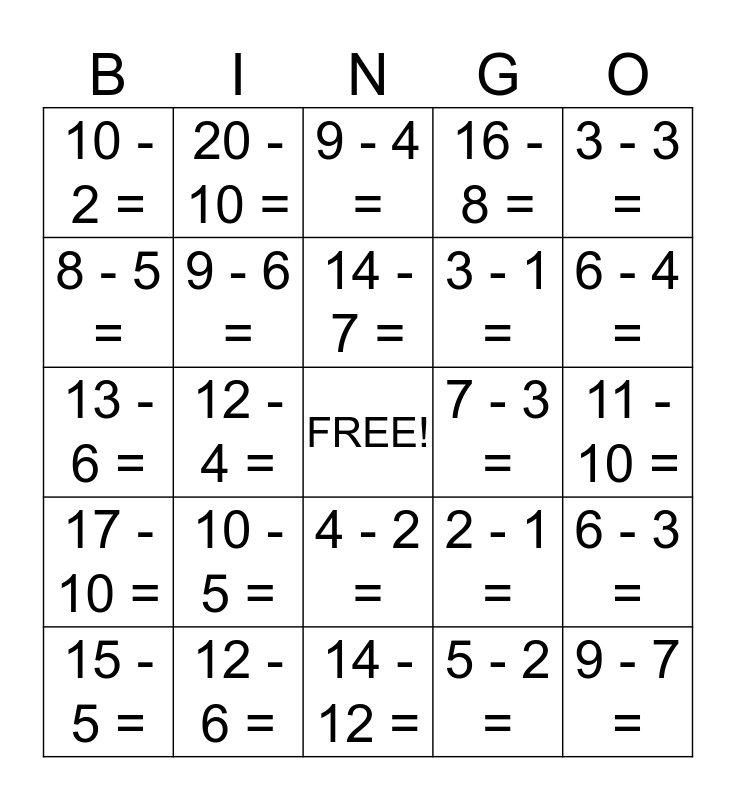# Subtraction BINGOThis bingo card has a free space and 24 words: 10 - 5 =, 12 - 6 =, 14 - 7 =, 15 - 5 =, 3 - 3 =, 10 - 2 =, 8 - 5 =, 20 - 10 =, 7 - 3 =, 4 - 2 =, 3 - 1 =, 13 - 6 =, 2 - 1 =, 6 - 3 =, 9 - 6 =, 5 - 2 =, 11 - 10 =, 9 - 4 =, 6 - 4 =, 12 - 4 =, 9 - 7 =, 17 - 10 =, 16 - 8 = and 14 - 12 =.

## Play Online